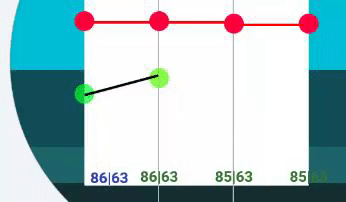# [PROBLEM] Simple Line Between Point A and Point B

Hello there!

Here is my Math (?) problem:

I am trying to join two points with a line - sounds easy, however I am not really able to find a solution I could be 100% satisfied right now.

Before I tried ;), I was sure, it must work with the Pythagoras’s theorem and a Sinusoid function.

To be sure, that the theory is working at different angles I prepared two simple moving points connected with the dynamic changed tag #BLN# up and down:

Than, I feeded the system with expressions:

Point A (Green left)

Y: (260-((100-#BLN#)*1.6))

Point B (Green right)

Y: (260-((#BLN#)*1.6))

LINE (Black)

`Y: (260-((100-#BLN#)*1.6))`

(Simple Pythagoras’s theorem – „60” is the horizontal distance and it does not change)

Width: (sqrt((60*60)+((((100-#BLN#)*1.6)-(#BLN#1.6))(((100-#BLN#)*1.6)-(#BLN#*1.6)))))

&

Rotation: (deg(2sin(((100-#BLN#)-#BLN#)/(sqrt((6060)+((((100-#BLN#)*1.6)-(#BLN#1.6))(((100-#BLN#)*1.6)-(#BLN#*1.6))))))))

Just for a better orientation, here is the same with color brackets as an image:

And the result:As you see, the dynamic changed width (Black Line) seems to be OK, but the angle (Rotation) is just flying around the B point (green point at right). The goal is to prepare a forecast line diagram (I found yesterday only one such a watchface at facer.io*).

My final question: Where did I make a mistake?

I am sorry I could not find this watchface right now (I am not on my computer). However this design shows two lines for low and high temprature for the next app. 5 days. What I remember more is, that the creator war a premium designer*.

**UPDATE (Dec 27, 2017): Here is the watch face from @eradicator09 (tr0n09) in real I was talking about:

2 Likes

Oh boy…

Our love-hate relationship with math…

thanks for your comment and the link to www.wolframalpha.com … I do have another possible solution(s) in mind*, however I was curious, why the (almosts) simplest formulas do not work properly when I am using it